# Java Program to Find Greatest Number

In this tutorial you will learn about the Java Program to Find Greatest Number and its application with practical example.

## Java Program to Find The Greatest Number

In this tutorial, we will learn to create a Java program that will Find The Greatest Number using Java programming.

## Prerequisites

Before starting with this tutorial, we assume that you are the best aware of the following Java programming topics:

• Operators in Java Programming.
• Basic Input and Output function in Java Programming.
• Basic Java programming.
• Conditional statement in Java programming.

## Program to Find the Greatest Number

In Java programming, it is possible to take multiple integer inputs from the user and Find the Greatest Number with the help of a very short program. The Java language has many types of header libraries which has supported function in them, with the help of these files the programming is easy. But today we will Find the Greatest Number from the taken numbers from the user using Java programming.

## Algorithm:-

With the help of this program, we can Find the Greatest Number.

## Output:-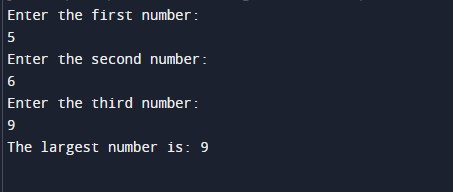In the above program, we have first initialized the required variable.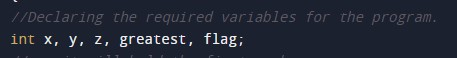• x= it will hold the first integer value.
• y = it will hold the second integer value.
• z = it will hold the third integer value.

Input numbers for the program.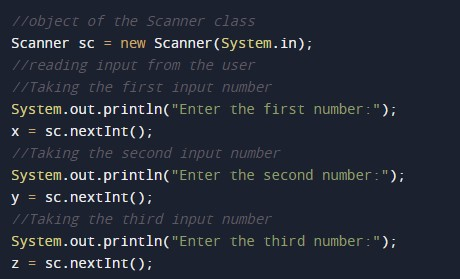Program Logic Code.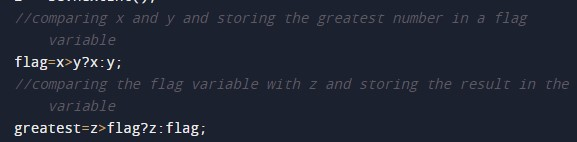Printing output for the greatest number.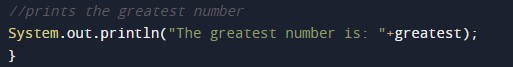In this tutorial we have learn about the Java Program to Find Greatest Number and its application with practical example. I hope you will like this tutorial.